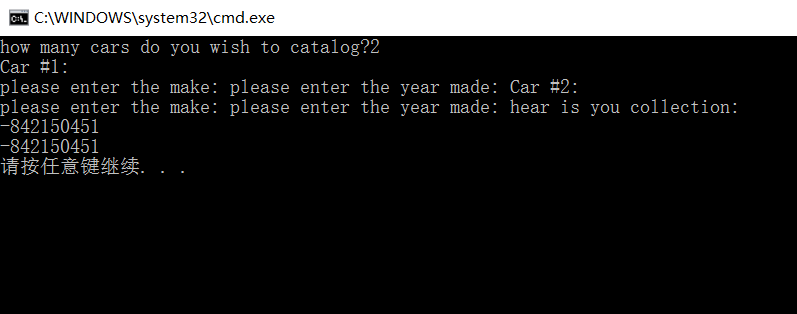`````` #include<iostream>
#include<ctime>
using namespace std;

struct Car
{
char name;
int year;
};
int main()
{
int num;
//int ch;
cout << "how many cars do you wish to catalog?";
cin >> num;
//ch=cin.get();
//cout << ch << endl;
//while (cin.get() != '\n');
Car *car = new Car[num];
for (int i = 0; i < num; i++)
{
cout << "Car #" << i + 1 << ":" << endl;
cout << "please enter the make: ";
cin.get(car[i].name,500);
//cin.get();
//cin.getline(car[i].name, 500).get();
cin >> car[i].year;
//cin.get();
//while (cin.get() != '\n');
}
cout << "hear is you collection: " << endl;
for (int i = 0; i < num; i++)
cout << car[i].year << " " << car[i].name << endl;
delete[]car;
return 0;
}
``````6个回答

`````` struct Car
{
char name;
int year;
};
int main()
{
int num;
//int ch;
cout << "how many cars do you wish to catalog?\n";
cin >> num;
//ch=cin.get();
//cout << ch << endl;
//while (cin.get() != '\n');
Car *car = new Car[num];
for (int i = 0; i < num; i++)
{
cout << "Car #" << i + 1 << ":" << endl;
cout << "please enter the make: ";
cin >> car[i].name;
//cin.get();
//cin.getline(car[i].name, 500).get();
cin >> car[i].year;
//cin.get();
//while (cin.get() != '\n');
}
cout << "hear is you collection: " << endl;
for (int i = 0; i < num; i++)
cout << car[i].year << " " << car[i].name << endl;
delete[]car;
return 0;
}
``````

#include

#include
#include
using namespace std;

struct Car
{
char name;
int year;
};
int main()
{
int num;
cout << "how many cars do you wish to catalog?";
scanf("%d",&num);
Car *car = new Car[num];
for (int i = 0; i < num; i++)
{
cout << "Car #" << i + 1 << ":" << endl;
cout << "please enter the name: ";
scanf("%s",car[i].name);
car[i].year=cin.get();
scanf("%d",&car[i].year);

``````}
cout << "hear is you collection: " << endl;
for (int i = 0; i < num; i++)
cout <<"Car #" <<i+1<<" "<<car[i].year << " " << car[i].name << endl;
delete[]car;
return 0;
``````

}

cin.get(car[i].name,500);//获取长度为500的文件流？？
//cin.get();//先获取文件流
//cin.getline(car[i].name, 500).get();//然后根据行将文件流读取到字符数组中
//所以第二种方法应该没问题！！

istream& get (char* s, streamsize n);

Extracts characters from the stream and stores them in s as a c-string, until either (n-1) characters have been extracted or the delimiting >character is encountered: the delimiting character being either the newline character ('\n') or delim (if this argument is specified).
The delimiting character is not extracted from the input sequence if found, and remains there as the next character to be extracted from the stream (see getline for an alternative that does discard the delimiting character).
A null character ('\0') is automatically appended to the written sequence if n is greater than zero, even if an empty string is extracted.
istream

``````  for (int i = 0; i < num; i++)
{
cout << "Car #" << i + 1 << ":" << endl;
cout << "please enter the make: ";
cin.get();
cin.get(name,500,'\n');
cin.get();